# Divine Proportions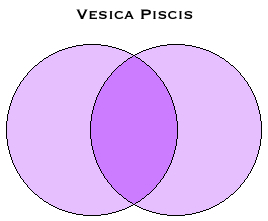The vesica piscis is an elegant figure made from two circles of equal size, intersecting so that each touches the centre of the other. Mathematically it is an interesting object, incorporating the square roots or 2, 3 and 5, and of course pi from its generating circles. The central oval is called a vesica and has been variously likened to a fish, lens, eye, mouth, almond nut, vagina and bladder (the words 'vesica piscis' mean 'fish bladder' in Latin). In Christian art an aureola in the shape of a vesica, called a mandorla (after the Italian for almond nut), often surrounds depictions of Christ or the Virgin Mary.

MANDORLAUsing simple mathematical operations and the standard value system of gematria, applied to familiar words and phrases in bibical Hebrew, biblical Greek and modern English, I will show that the proportions of the vesica piscis and of the circle itself are imprinted onto divine nomenclature. The geometric element this time comes from classical plane geometry.

Proportional Representation

The linear dimensions of the vesica piscis relative to the circle are often quoted. However, at first I would like to concentrate on its area relative to the circle. This is shown below, based on the circle with an area of 1000 square units (the unit area of 1 times 1000, which gives a suitable quantity to work with). All given areas are accurate to at least three significant figures.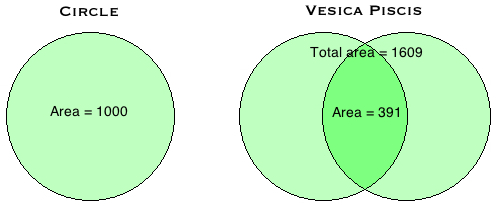So for a circle area of 1000 units, the total area of the intersecting circles is 1609 units and the area of the vesica formed is 391 units. When multiplying and dividing whole numbers by pi it is impossible to obtain a whole number as a result, but it is worth noting here that if we work out the areas of the vesica and the intersecting circles with more precision we obtain 391.002 and 1608.998, extremely close to the whole numbers 391 and 1609.

These are the standard values of the following names: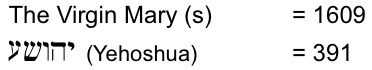The traditional depictions of Jesus and the Virgin Mary within a mandorla are reflected in this very special vesica piscis, where again the Virgin gives birth to the Christ! Given the almond shape of the vesica itself, it is surely also significant that Aaron's Staff that Had Budded, held within the Ark of the Testimony, and a symbol of the Messiah's authority, was thought to be from an almond tree (Numbers 17.8).

The circle area of 1000 units is equal to the volume of the Most Holy Place of the Tabernacle, which was a perfect cube 10 cubits on a side. Like the circle, the cube symbolises divine perfection and the Most Holy Place was the symbolic womb within which the Ark of the Testimony was kept in the Tabernacle. Thus the connection here to the birth of Christ resonates strongly with the New Bible Code's interpretation of 9/11 as the ultimate Day of Atonement and the conception of the Male Child.

The numbers 391 and 1609 practically define this vesica piscis, and a third defining number is 1218, the sum of the two touching crescents of 609 units either side of the vesica. Seeing 391, 1218 and 1609 together in the text would be a strong indication that this particular figure is being encoded. In fact all three numbers are found in close proximity within the first few verses of the NIV Bible and are part of what I now call the Genesis Watermark, a holographic imprint miraculously placed upon its first five verses. Here are the encodings. I've also calculated the probability of their occurrence, including 1000 among the search numbers, as it is the area of the generating circle.

These calculations are based on the simple technique of summing the ordinal values of consecutive words, starting from word 1, until the desired numbers are either skipped over or landed upon. The calculation is then begun again starting from word 2, then from word 3, etc. This technique ensures that every possible word string is numerated and naturally lends itself to probability calculations using the binomial formula.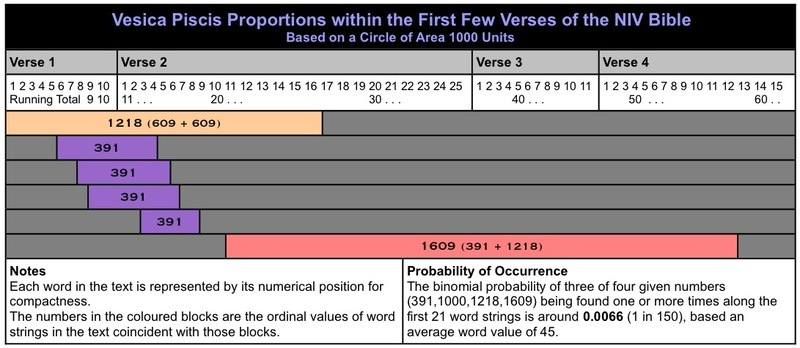It is undoubtedly significant that the first two numbers encoded are 1218 and 391, which complete the vesica piscis. All of these numbers are encoded close by and in fact 391 is found six times in the first 37 words, at odds of 8000 to 1 against. 391 is perhaps the most significant number in the code, the value of 'Yehoshua' in Hebrew and 'Second Coming' in English!

Ever-Increasing Circles

By increasing the number of circles involved, we can produce intersections geometrically related to the vesica piscis. Some of these have areas that are also found as gematria related to Jesus Christ.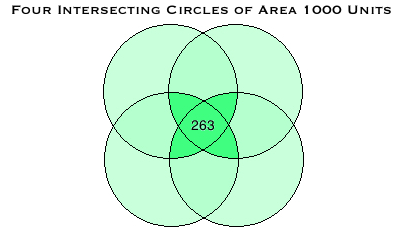Messiah (s) = 263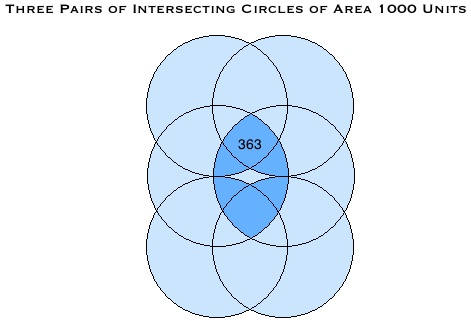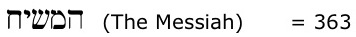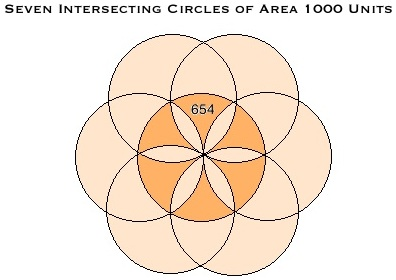Word (s) = 654

This final figure is called the Seed of Life and is the precursor of the Flower of Life, an ancient and fundamental geometric pattern.

Drawing the Line

If we now calculate the circumference of the starting circle (area 1000 units), we make another divine connection, with the Hebrew words Yahweh Elohim, translated as 'The Lord God' and first found in Genesis 2.4.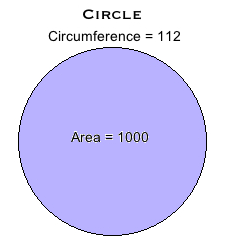The circumference of the vesica obtained from this circle is 75 units, the ordinal value of 'Lord God', and the total circumference of both intersecting circles, including the vesica, is 224, the value of an alternative spelling of Ruach, Hebrew for 'Spirit'.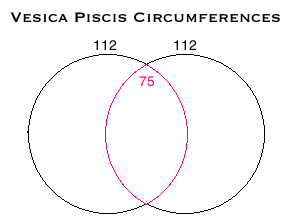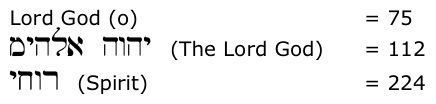Sizing Up the Circle

We can begin with a circle of any size, of course, and if we create a circle such that the vesica made by the intersection of two such circles has a half circumference of 391 units. This is an arc of the circle itself and the diameter of that circle is 373 units. The number 373 is the gematria of the Greek word logos, meaning 'Word'.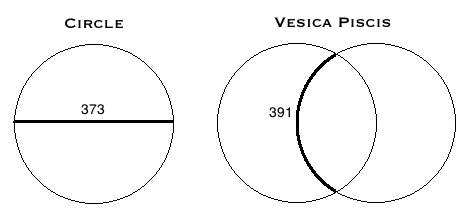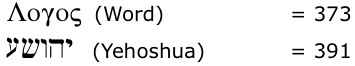So the proportions of the vesica piscis link the names 'Yehoshua' and 'Logos' and support the opening statements in the Gospel of John that Jesus is the Word made flesh (John 1.14).

The Greek New Testament mostly uses the name Ihsous Christos for Jesus Christ, with gematria 2368. If we construct two circles, one with a circumference of 2368 units, and the other with an area of 2368 square units, we find that the Hebrew and English names for Jesus Christ are automatically generated. The names in all three langauges, Ihsous Christos, Yehoshua HaMashiach and Jesus Christ, along with their numerical values under the standard value system of numeration, applied in the same way to all three alphabets, is given below.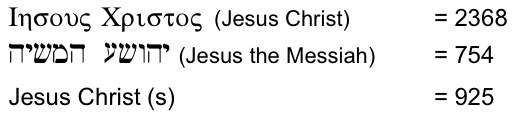Both 754 and 925 can be derived from 2368 by making 2368 the circumference of a circle then calculating the diameter, and by making 2368 the area of a circle then creating a vesica piscis from it.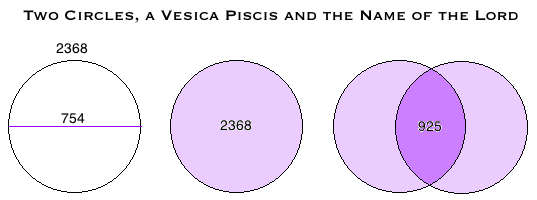Note: The area of the vesica is 925.8, which should actually round up to 926. Because it is otherwise such a compelling relationship, supported by the other numerical relationships detailed here, and because there is a tradition of colel in Kabbalah, meaning that plus or minus one digit is allowed, since "each number contains the seed of the next". I have accepted this mathematical relationship as supporting evidence for the reality of the New Bible Code. Readers can decide for themselves whether they agree.

Summary

Once again we see the nomenclature of the divine embodying fundamental mathematical truths. The form of the vesica piscis and its proportions, built into the names and titles by which we know Jesus Christ have a symbolic message for us. The intersecting circles portray the same image as the intersecting triangles in a Star of David, that of heaven meeting earth in the Son of God.

Bill Downie 13/4/15